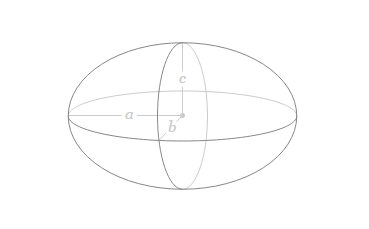# Volume of an Ellipsoid Formula

An ellipsoid is a closed quadric surface that is a three-dimensional analogue of an ellipse. The standard equation of an ellipsoid centred at the origin of a Cartesian coordinate system. The spectral theorem can again be used to obtain a standard equation akin to the definition given above.The formula of Ellipsoid is given below:

$\large V=\frac{4}{3}\pi\,a\,b\,c$

or the formula can also be written as:

$\large V=\frac{4}{3}\pi\,r_1\,r_2\,r_3$

Where,
a = r1 = Radius of the ellipsoid of axis 1
b = r2 = Radius of the ellipsoid of axis 2
c = r3 = Radius of the ellipsoid of axis 3

### Volume of an Ellipsoid Formula Solved Example

Example: The ellipsoid whose radii are given as a = 9 cm, b = 6 cm and c = 3 cm. Find the volume of an ellipsoid.

Solution:

Given,
$$\begin{array}{l}V=\frac{4}{3}\pi\,a\,b\,c\end{array}$$
$$\begin{array}{l}V=\frac{4}{3}\times 3.14\times9\times6\times3\end{array}$$
$$\begin{array}{l}V=678.24\,cm^{3}\end{array}$$